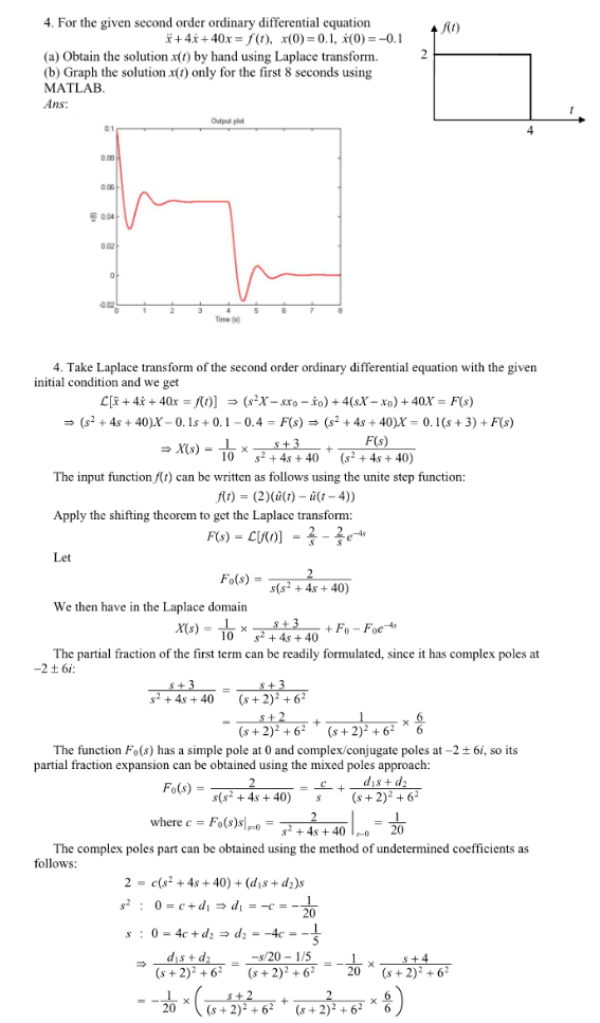For the given second order ordinary differential equation, xdoubledot+4xdot+40x=f(t), x(0)=0.1, xdot(0)=-0.1, (a) Obtain the solution x(t) by hand using Laplace transform, (b) Graph the solution x(t) only for the first 8 seconds using MATLAB.For the given second order ordinary differential equation, xdoubledot+4xdot+40x=f(t), x(0)=0.1, xdot(0)=-0.1, (a) Obtain the solution x(t) by hand using Laplace transform, (b) Graph the solution x(t) only for the first 8 seconds using MATLAB.

System Dynamics Page 2 dynamics dynamics dynamics dynamics dynamics dynamics dynamics System dynamics Page 3